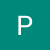# Foundations of Data Science

Nowadays, all of us have heard that “Data is the new oil and the Data Science is the combustion engine that drives it” or “Data Science is the most sought after job of the twenty-first century” or “Data Science is the future”. …

# Operations on NumPy arrays

In the previous article, we discussed how to index the NumPy arrays. In this article, we discuss how to perform operations on NumPy arrays. Let’s get started.

Let’s create some sample arrays of the same size to play around with, the good thing with NumPy is that we can treat…

# Indexing NumPy arrays

In the last article, we discussed how to create NumPy arrays. In this article, we discuss how to index different slices of the NumPy array i.e access different parts of the ‘n-dimensional’ array.

Say we have a 3D array:

# High dimensional array and Creating NumPy array

In the last article, we started with the NumPy package and discussed its advantages, the performance it offers. In this article, we discuss how to create a NumPy array.

Let’s just visualize what the high-dimensional array looks like. …

# How to describe relationships between variables?

In the last article, we discussed how a histogram could help us understand the data distribution for an attribute. In this article, we discuss how to understand the relationship between multiple attributes.

# Scatter Plots

This is a common observation in many real-world datasets where for a given object, we have several attributes…

# Stem and Leaf plots

In the last article, we looked at the histogram as a way of describing and understanding the trends in Quantitative Data. Let’s look at another kind of plot for describing Quantitative Data which is the Stem and Leaf plot. …

# Uses of Histograms in Machine Learning

In the last few articles, we have discussed histogram in great detail, and it's clear by now that histogram reveals a lot of trends in the data, it helps us understand interesting patterns in the data. Histograms are also used in Machine Learning for various purposes and in this article…

# Typical trends in Histogram

The main purpose when we draw a histogram is to see if some trends are visible in the data or not and there are some standard trends that we should look out for in the histogram. These standard trends are discussed in this article. So, let’s get started.

Here is…

# Histogram

In the previous article, we looked at how Histogram is the preferred way of representing Quantitative Data and how to select the perfect bin size. In this article, we look at how to answer questions on percentages /proportions— “In what percentage of matches did Sachin score less than 10 runs?”